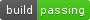# plotly

An R package for creating interactive web graphics via the open source JavaScript graphing library plotly.js.

## Installation

Install from CRAN:

``install.packages("plotly")``

Or install the latest development version (on GitHub) via devtools:

``devtools::install_github("ropensci/plotly")``

NOTE: The CRAN version of plotly is designed to work with the CRAN version of ggplot2, but at least for the time being, we recommend using the development versions of both plotly and ggplot2 (`devtools::install_github("hadley/ggplot2")`).

## Getting started

### Web-based ggplot2 graphics

If you use ggplot2, `ggplotly()` converts your static plots to an interactive web-based version!

``````library(plotly)
g <- ggplot(faithful, aes(x = eruptions, y = waiting)) +
stat_density_2d(aes(fill = ..level..), geom = "polygon") +
xlim(1, 6) + ylim(40, 100)
ggplotly(g)``````

By default, `ggplotly()` tries to replicate the static ggplot2 version exactly (before any interaction occurs), but sometimes you need greater control over the interactive behavior. The `ggplotly()` function itself has some convenient “high-level” arguments, such as `dynamicTicks`, which tells plotly.js to dynamically recompute axes, when appropriate. The `style()` function also comes in handy for modifying the underlying traces attributes used to generate the plot:

``````gg <- ggplotly(g, dynamicTicks = "y")
style(gg, hoveron = "points", hoverinfo = "x+y+text", hoverlabel = list(bgcolor = "white"))``````

Moreover, since `ggplotly()` returns a plotly object, you can apply essentially any function from the R package on that object. Some useful ones include `layout()` (for customizing the layout), `add_traces()` (and its higher-level `add_*()` siblings, for example `add_polygons()`, for adding new traces/data), `subplot()` (for combining multiple plotly objects), and `plotly_json()` (for inspecting the underlying JSON sent to plotly.js).

The `ggplotly()` function will also respect some “unofficial” ggplot2 aesthetics, namely `text` (for customizing the tooltip), `frame` (for creating animations), and `ids` (for ensuring sensible smooth transitions).

### Using plotly without ggplot2

The `plot_ly()` function provides a more direct interface to plotly.js so you can leverage more specialized chart types (e.g., parallel coordinates or maps) or even some visualization that the ggplot2 API won’t ever support (e.g., surface, mesh, trisurf, or sankey diagrams). The cheatsheet is a nice quick reference for this interface, but the plotly cookbook has more complete overview of the philosophy behind this “non-ggplot2” approach.

``plot_ly(z = ~volcano, type = "surface")``